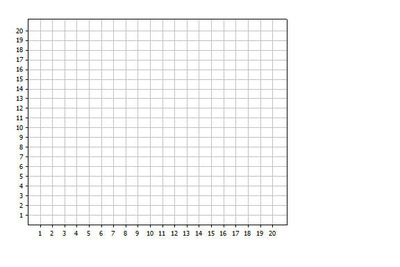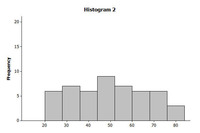# Understanding the Standard Deviation

Alignments to Content Standards: S-ID.A.2

This task is divided into four parts.

Â

Part 1

Below are dot plots for three different data sets. The standard deviations for these three data sets are given in the following table. Looking at the dot plots and without calculating the standard deviations, match the data sets to the standard deviations.

Â Â Â Â Â Â Â Â Â Â Â Â Â Â Â Â Â Â Â Â Â Â Â

 Standard deviation Data Set 5.9 Â 3.3 Â 2.3 ÂPart 2

Â

1. Draw 4 rectangles for which the standard deviation of the 4 rectangle heights would be equal to 0.1. Draw 4 rectangles for which the standard deviation of the 4 rectangle heights would be greater than the standard deviation of the rectangle widths.Â

Part 3

Which of the two histograms below represents the data distribution with the greater standard deviation? Explain your choice.

ÂÂ

Â

Part 4

1. Write two sets of 5 different numbers that have the same mean but different standard deviations.
Â

2. Write two sets of 5 different numbers that have the same standard deviations but different means.
Â

Â

## IM Commentary

The purpose of this task is to deepen student understanding of the standard deviation as a measure of variability in a data distribution. The task is conceptual rather than computational and does not require students to calculate the standard deviation.

The task is divided into four parts, each of which asks students to think about standard deviation in a different way. Parts 3 and 4 may be difficult for students, and you may want to let them work in groups to complete these parts.

If students struggle with Part 2, ask them what it means for the standard deviation to be equal to zero. What does this imply about the rectangle heights?

Part 3 addresses a common student error when thinking about the variability and how the standard deviation measures variability. Students often pick the incorrect histogram as the one for which the standard deviation would be largest because they are thinking about variability in the heights of the bars in the histogram and not understanding that the standard deviation measures variability in the variable that defines the measurement scale in the histogram.

If students struggle with Part 4, you might show them a set of five numbers and ask them what would happen to the mean if you were to add 5 to every number. Then ask if this would change the variability in the data. This will give them a way to think about how to complete part 4.

Â

## Solution

Part 1

 Standard deviation Data Set 5.9 3 3.3 2 2.3 1

Part 2

Answers will vary. For question 1, students should draw a set of 4 rectangles that all have the same height. The widths can vary, or they can all be the same. For question 2, the heights of the 4 rectangles drawn should vary more than the widths.

Part 3

The variability would be greater for the data set displayed in Histogram 2. The values in the data set are more spread out relative to the mean of the data set. The data values in Histogram 1 cluster more tightly around the mean of that distribution.

Part 4